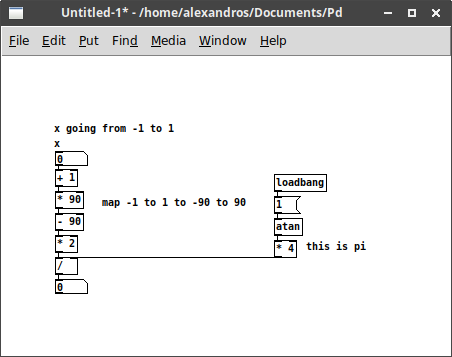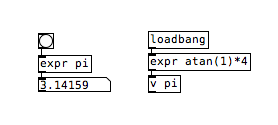• ### Wave Folding

Hello, I am super new to Pd but have been using Audulus for about a year. I was thinking about getting a guitari and critter organelle so I could load my own patches in a hardware device and thought I would see how well I could get on in Pd. I'm a lost at the moment, and while I plow my way through hours of tutorial videos I thought I might ask for a little help.

How do I send a waveform of varying amplitude through this expression in Pd?

2 * asin( sin( x *(pi/2)))/pi

• Posts 12 | Views 7426
• w00t! I made a basic patch, achievement unlocked• @robertsyrett Nice! I don't know how to convert Pi into something that expr calculates rather than using the super long number. Let's wait if somebody more knowledgeable can suggest a more elegant solution.

• "acos(-1)" and "atan(1)*4" might be close enough approximations

• @weightless "acos(-1)" might be more characters than "pi" but far far fewer than 3.1415......... And it still seems to work fine, thanks again!

• Trying out some things with this expression, it seems to me that all this part does `asin(sin(x *(pi/2)))` is to map values between -1 and 1 to a range between -90 and 90. Calculating this part `sin(x*(pi/2))` with values for x between -1 and 1, and using an arcsin calculator, for -1 I got -90, for -0.5 I got almost -45, for 0 I got 0, for 0.5 I got almost 45, and for 1 I got 90.
So, if you're using values for x between -1 and 1, you could probably replace this with a mapped version of x, and the whole thing could be written like this:Otherwise, if you want to use `[expr]`, you should save the 'pi' value to a `[value pi]` (also abbreviated `[v pi]`, where 'pi' is the argument), and then use it in `[expr]` with its variable name. Mind though that `[expr~]` is pretty CPU hungry, especially when it comes to hardware like the Organelle.

I might be wrong about the whole arcsin thing though...

• @alexandros would three variable wave oscillators and my wavefolder really overwhelm the organelle?

Yes, the idea of a wavefolder is to reflect the waveform when it exceeds the range of -1 to 1. So the plan is to send the wavefolder a waveform and offset that signal by a varying amounts and multiply it's amplitude many times so that it goes outside -1 to 1 and folds back on itself creating new harmonics. If you open the patch I made you can see what it does. A very similar wavefolder would be sin( \$v1 * asin(1)) or even cos (x) but I like 2 * asin (sin( \$v1 * (pi/2)))/pi because it has more harmonics.

I would love it if you could upload a patch of your atan concept, I'm still hungry to learn how Pd works and practical examples I can relate to would be welcome.

• @robertsyrett this was his cleaver suggestion on how to use "pi" inside exprthe object [value] (also called [v]) lets you store a float (like [float]) but you can give it a name and use that name inside [expr] to recall that value. Just make sure the name doesn't contain mathematical symbols like + - . etc.. and also the name can't start with a float, so calling a variable "1pi" won't work, whereas "pi1" will. This is important if you need the name to include \$0 (which is internally converted to a unique number for each instance of the patch), remember not to put \$0 at the beginning of the name (so you would use pi\$0).

Hope this makes sense.

• @weightless It is starting to. That is a very different paradigm than Audulus. If I had a patch with multiple wave folders would each one need to have a separate variable, like \$v1 in one and \$v2 in the other?

• @robertsyrett You can use different variables inside an expr object, and they refer to the corresponding inlets. For example if you have [expr~ \$v1 + \$v2 + \$f3], the object will have 3 inlets, the first two are signal inlets (\$v refers to a signal) and the third will be a control inlet (\$f refers to a float). The help file for [expr] is very useful for that, and you can find further information here http://yadegari.org/expr/expr.html

When you say different wavefolders, I would assume you mean different equations as the [expr~ asin(sin(\$v1 *(pi/2)))] object is itself the wavefolder. The \$v or \$f variables refer to the object's inlets.

Posts 12 | Views 7426
Internal error.

Oops! Looks like something went wrong!